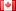# Revision history [back]

### Polinomial: find the common factor

Hello, assuming I have a polinomial like this:

sage: var('y, x')
(y, x)
sage: y = x^2 + 6*x^3 + 3*x^4


When I use collect_common_factors(), I get:

(3*x^2 + 6*x + 1)*x^2


Now, is there a way to know which are the common_factors collected?

In this case, I'm expecting this output:

x^2


Thank you everyone!2 No.2 RevisionDSM5232 ●21 ●68 ●112

### Polinomial: Polynomial: find the common factor

Hello, assuming I have a polinomial polynomial like this:

sage: var('y, x')
(y, x)
sage: y = x^2 + 6*x^3 + 3*x^4


When I use collect_common_factors(), I get:

(3*x^2 + 6*x + 1)*x^2


Now, is there a way to know which are the common_factors collected?

In this case, I'm expecting this output:

x^2


Thank you everyone!3 No.3 RevisionDSM5232 ●21 ●68 ●112

### Polynomial: find the common factor

Hello, assuming I have a polynomial like this:

sage: var('y, x')
(y, x)
sage: y = x^2 + 6*x^3 + 3*x^4


When I use collect_common_factors(), I get:

(3*x^2 + 6*x + 1)*x^2


Now, is there a way to know which are the common_factors collected?

In this case, I'm expecting this output:

x^2


Thank you everyone!4 retaggedFrédéricC4131 ●3 ●37 ●85

### Polynomial: find the common factor

Hello, assuming I have a polynomial like this:

sage: var('y, x')
(y, x)
sage: y = x^2 + 6*x^3 + 3*x^4


When I use collect_common_factors(), I get:

(3*x^2 + 6*x + 1)*x^2


Now, is there a way to know which are the common_factors collected?

In this case, I'm expecting this output:

x^2


Thank you everyone!